# Digging into convolution

Feb 28, 2020 · 4 mins readIssues

1) Kaiming Initializtion in Pytorch was in trouble.1

2) Jeremy started to dig in, in lesson09, but I didn’t know why the size of tensor is 2 and even understand this spreadsheet data.3

### What is a convolution?

A convolutional neural network is that your red, green, and blue pixels go into the simple computation, and something comes out of that, and then the result of that goes into a second layer, and the result of that goes into the third layer and so forth.

#### Visualization

##### one kernel
• Refer this site for visualizing CNN filtering
##### Matthew D Zeiler & Rob Fergus Paper

Lecture01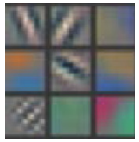#### Convolution can be represented as matmul

CNNs from different viewpoints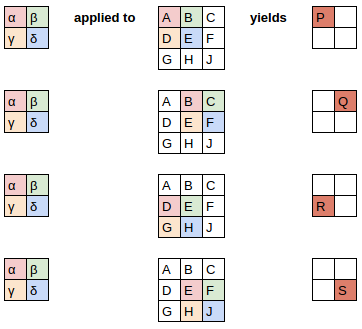{align-items: center;}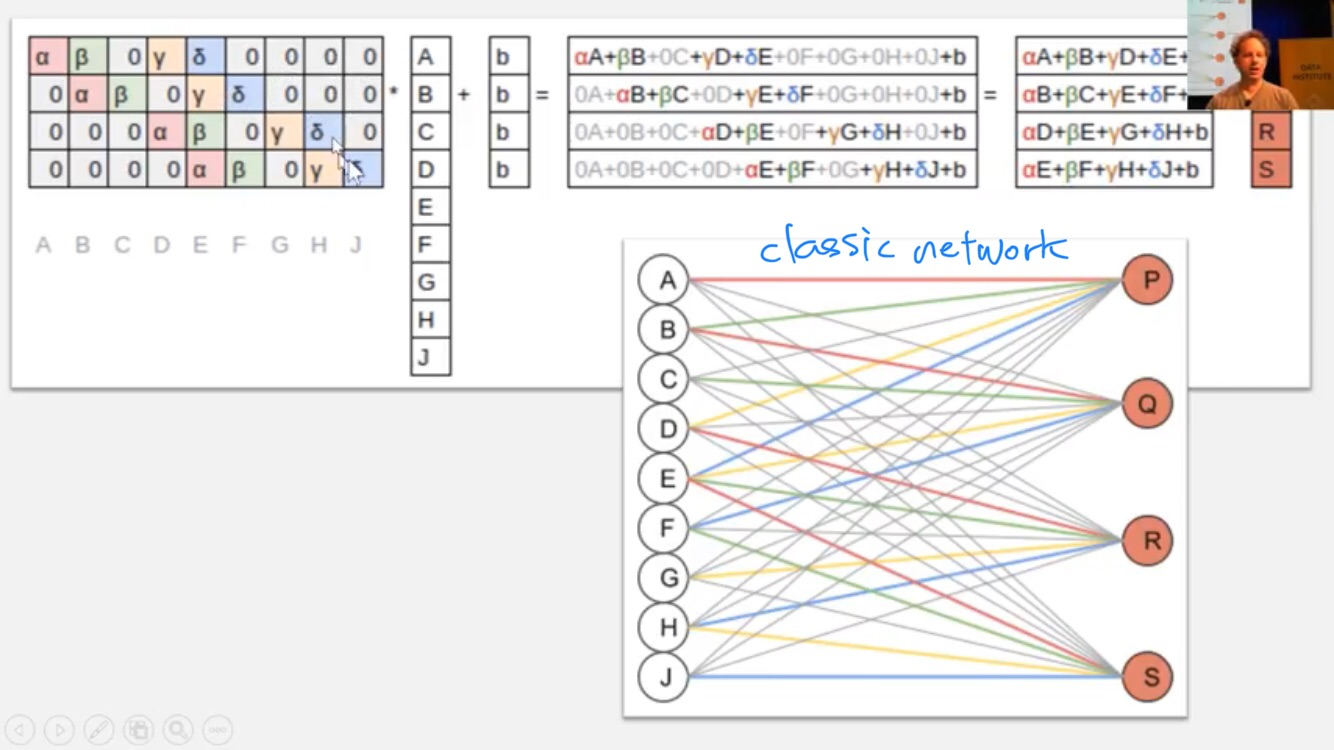• [A B C D E F G H I J] is 3 by 3 image data flatten to vector.

• As a result, convolution is a just matrix just two things happens
• Some of entries are set to zeros at all the times
• same color always have the same weight. That called weight time / wegith sharing
• So, we can implement a convolution with matrix multiplication. But, we don’t do that because it’s slow!

• What most of libraries do is just put zeros asdie of matrix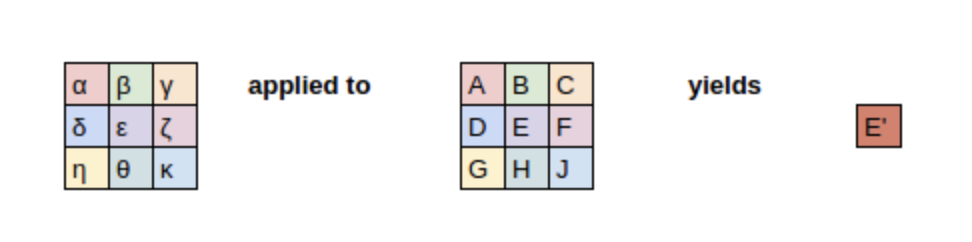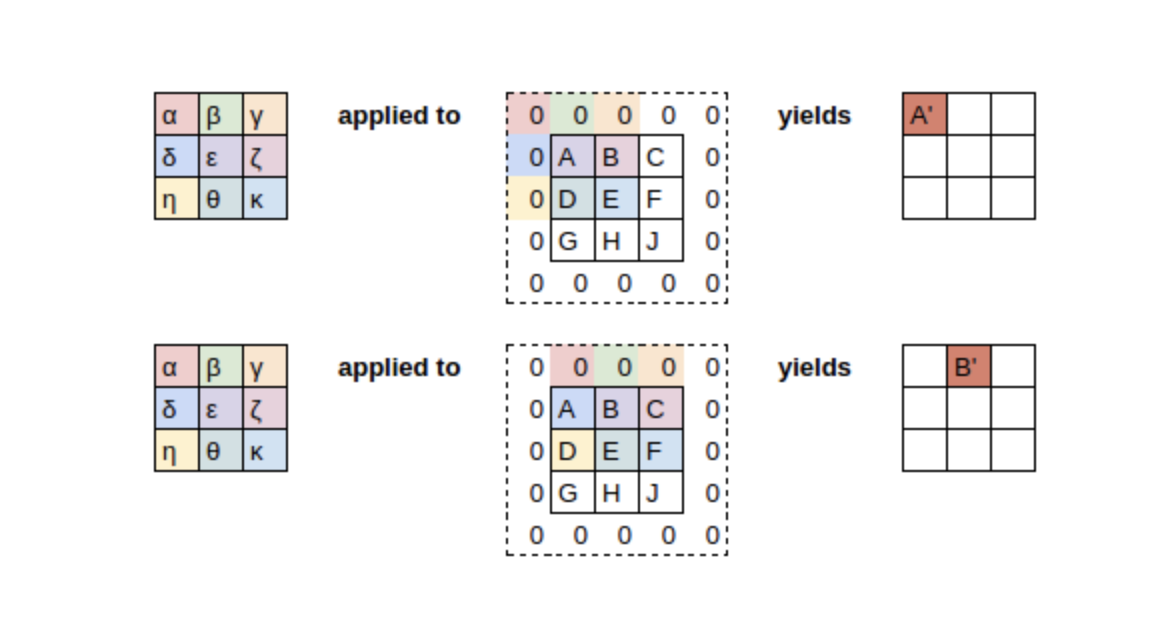• fast.ai uses reflection paddings (what is this? Jeremy said he uttered it)

#### Kernel has rank 3

• As standard picture input would be 4 5, it would be actually 3d, not 2d.
• If we make kernel as a 3x3 size, we pass over same kernel all the different Red, Green, Blue Pixels.
• This could make problem, because, if we want to detect frog, which is green, we would want more activations on the green(I made a test cell in my colab 6)

#### How can we find a side-edge, a gradient and area of constant weight?

Not top-edge!

• One kernel can find only the top-edge, so we should stack the kernels 7
• So, we pass it through bunch of kernels to the input images, and that process gives us height x width x corresponding number of kernels.• Usually that number of chanel is 16
• And if we want to get the more channels and features, we should repeat that process
• This process gives rise to memory out of control, we do the stride

####

conv-example.xlsx

• 2 convolutional filters
• At a second layer, filter is 3x3x2 tensor, because to add up together the first layer’s channel.

### Reference

1. Problem was math.sqrt(5) was not kaiming initialization formula, Implementation in Pytorch

2. Grayscale is a group of shades without any visible color. … Each of these dots has its own brightness level as well and, therefore, can be converted to grayscale. A grayscale image is one with all color information removed.